﻿﻿ Current Induced in Wire-Wound Inductorexamples｜products｜Murata Software Co., Ltd.# Example27Current Induced in Wire-Wound Inductor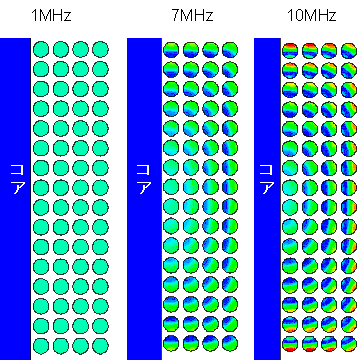### General

• The current induced in a wire-wound Inductor is analyzed.

• The current distribution and the resistance are solved.

• This will be an axisymmetric analysis.

• Unless specified in the list below, the default conditions will be applied.

### Analysis Space

 Item Settings Analysis Space Axisymmetric Model unit mm

### Analysis Conditions

 Item Settings Solvers Magnetic Field Analysis [Gauss] Analysis Type Harmonic analysis Options Deselect Inductance Calculation.

The frequency is swept from 1MHz to 10MHz in 1MHz interval.

 Tab Setting Item Settings Harmonic analysis Frequency Minimum: 1×10^6[Hz] Maximum: 10×10^6[Hz] Step Linear step: Division number: 9

### Model

The axisymmetric analysis is performed. For a model which is symmetric around Z axis,

the simulation is done for the X-Z section only. It is virtually 2D analysis.

The calculation is much faster than 3D analysis. The axisymmetric analysis is well suited for this exercise.

A core is placed in center, and the coil is wound on the core.

The coil is formed by 16 turns in Z direction and 4 layers in X direction. It is 64 turns in total.

The right half section of the model is analyzed.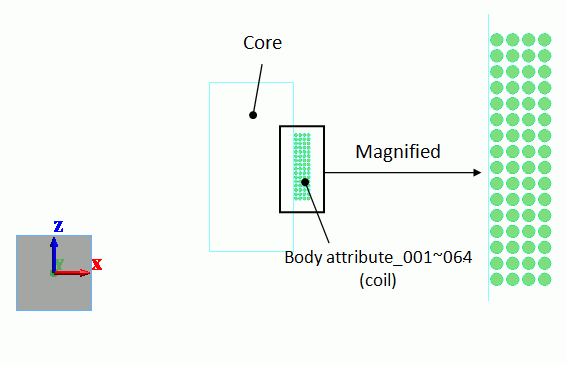### Body Attributes and Materials

 Body Number/Type Body Attribute Name Material Name Mesh Size 0-63/Sheet 65/Sheet Body_Attribute_001 to 064 CORE 008_Cu * CORE 0.01

* Available from the Material DB

Set the current as follows.

 Body Attribute Name Tab Settings Body_Attribute_001 to 064 Current Waveform: AC Current: 1[A] Turns: 1[Turns] Direction: +Y direction

No setting.

### Results

The following are solved.

1) Magnetic flux lines

2) Current density distribution

3) Frequency characteristics of the inductor’s resistance

4) Inductance

1) Magnetic flux lines

The figure below shows the magnetic flux lines at 10MHz.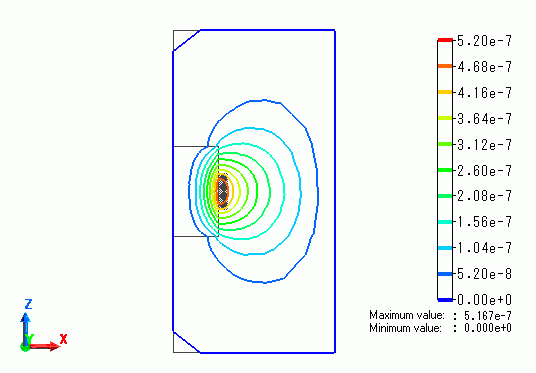2) Current density distribution

The figure below shows the current distribution. The current flows uniformly at 1MHz. At higher frequencies, the current flows unevenly especially in the outer windings.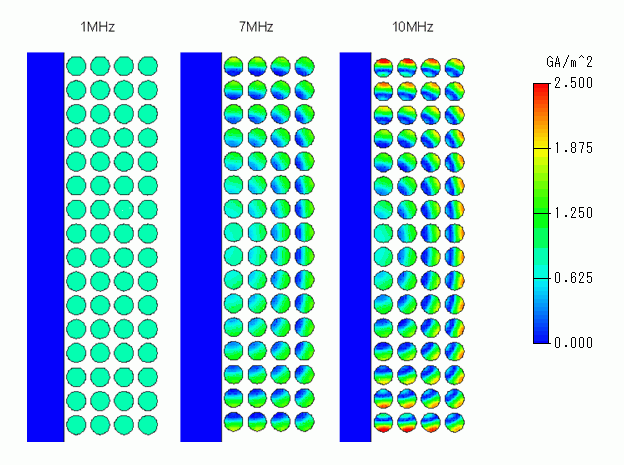3) Frequency characteristics of the inductor’s resistance

The chart below is the inductor’s resistance. It is higher at higher frequency.

• How to calculate the resistance
Resistance R can be calculated from the loss P [W] displayed on the output window:
R = 2*P, where P = 1/2*R*I*I and I = 1[A] peak.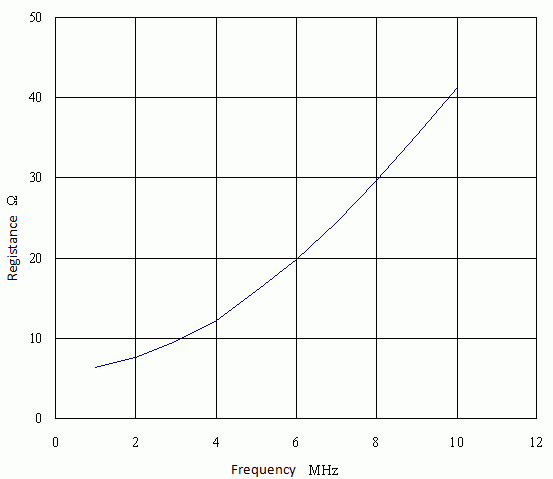4) Inductance

The inductance can be calculated as follows. The magnetic energy Em, the current I and the inductance L satisfy

Em = 1/2*L*I*I As Em = 1.563e-5[J] is obtained at I = 1[A] through the simulation,

L = 31.26[uH]

﻿ ﻿